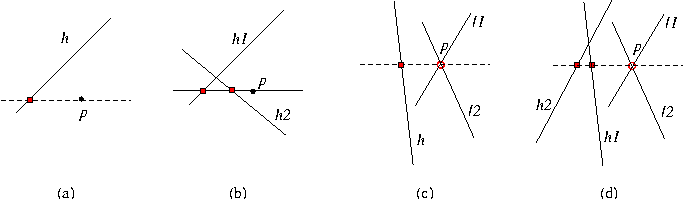## Kernel::CompareXAtY_2

A model for this must provide:

 Comparison_result fo.operator() ( Kernel::Point_2 p, Kernel::Line_2 h) compares the x-coordinates of p and the horizontal projection of p on h; see (a) in the figure below. Comparison_result fo.operator() ( Kernel::Point_2 p, Kernel::Line_2 h1, Kernel::Line_2 h2) compares the x-coordinates of the horizontal projection of p on h1 and on h2; see (b) in the figure below. Comparison_result fo.operator() ( Kernel::Line_2 l1, Kernel::Line_2 l2, Kernel::Line_2 h) Let p be the intersection of lines l1 and l2. This function compares the x-coordinates of p and the horizontal projection of p on h; see (c) in the figure below. Comparison_result fo.operator() ( Kernel::Line_2 l1, Kernel::Line_2 l2, Kernel::Line_2 h1, Kernel::Line_2 h2) Let p be the intersection of lines l1 and l2. This function compares the x-coordinates of the horizontal projection of p on h1 and on h2; see (d) in the figure below.